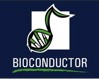# Statistical Consulting

BNOSAC consultants are experts in statistical consulting and biostatistical analysis. From adhoc analysis to integrated solutions.

StatisticsBNOSAC experts give consulting for the use of statistical modelling within commercial environments. We either do adhoc analysis or automated statistics and are specialised in the following statistical topics:

• Basic statistics
• Multivariate modelling (clustering, PCA, generalized linear models) and categorical data analysis
• Stochastic Simulations
• Predictive modelling: boosting, bagging, GAM, randomforests, SVM, neural networks and regularization techniques
• Mixed models, survival analysis, latent variable models
• Time series, state space models, filtering techniques, markov switching models and basic risk analysis
• Point pattern analysis and network analysis
• Data fusion techniques

BiostatisticsBNOSAC consultants have worked in biostatistical projects for several years, using SAS and working with hierarchical models and survival analysis. Our expertise domain in biostatistics is the following:

• Sample size and power calculations
• Design of Experiments
• Statistical analysis for clinical trials.
• Automation of reports
• Mixed modelling
• Bayesian analysis
• Survival analysis
• Matching
• Help in the use of bioconductor packages